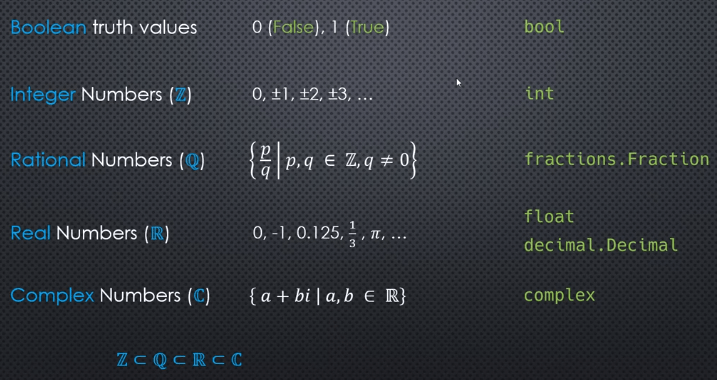## Automatic Semicolon Insertion

Anywhere the syntax parser expect that semicolon will be, it will put one for you, that why semicolons seems optional, as you’re writing the code, you should always put your own semicolons, also, automatic semicolon insertion could cause big problem

by avoid this mistake, you should always put curly brackets on the same line as functions, for loops and if statements etc. instead of put them one the newline.

## Numeric in Python

### 5 types### int

integers are represented using base-2(binary) digits, not base-10(decimal)

10011(base-2) required 5 bits

##### what’s the range of integer number can be represented using 8 bits?

11111111(base-2) required 8 bits, could represent largest 255

Using 8 bits are able to represent range [-127, 127], since 0 does not require a sign, we can squeeze out an extra number to [-128, 127]

How large an integer can be depends on how many bits are used, some languages(Java, C) provide multiple distinct integer types that use a fixed number of bits. Python doesn’t work that way, the int object uses a variable number of bits, can use 32, 64, 96 bits etc.(increase bits by need)

##### int arithmetic
• int +(-, *, **, //, %) int => int
• int / int => float

### Fraction

represent rational number

### float

represent real number

CPython float is implemented using the C double type which implements the IEEE double-precision binary float, also called binary64

float uses a fixed number of bytes, in standard, CPython/3.6/64bits using 8 bytes(64 bits)##### representation
• decimal• binaryproblem: some numbers do not have a finite representation

• 1/3 can not represent accurate by decimal expansion
• 1/10 can not represent accurate by binary expansion##### Coercing float to integer -> data loss

truncation: math.trunc(), constructor of int() use truncation

floor: math.floor()

ceiling: math.ceil()

round: round()

## 伊凡．伊里奇之死

「死亡結束了，」他對自己說：「再也沒也死亡。」